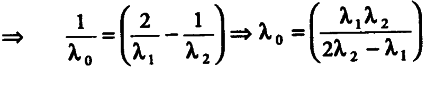# Write Einstein’s photoelectric equation

Write Einstein’s photoelectric equation and mention which important features in photoelectric effect can be explained with the help of this equation.The maximum kinetic energy of the photoelectrons gets doubled when the wavelength of light incident on the surface changes occurs. Derive the expressions for the threshold wavelength and work function for the metal surface

According to the photoelectric equation